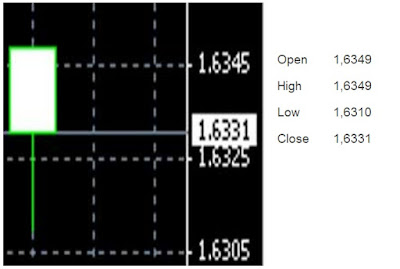How to Use Pivot Points in Forex Trading - ForeXposed

# ForeXposed

Exposing Forex World

## Wednesday, September 13, 2017

In this article, we will discuss other aspects in forex trading. We will study chart and began moving into the fundamental analysis. And this time we will address a session called Pivot Points!

This material is actually not too interesting to be discussed. But somehow, many forex traders still ask what it's pivot points. May indeed be useful. So, we felt it necessary to include it in the series of our writing, due to the many requests, from time to time.

The Pivot Points calculation is a way to determine the areas of support andresistance. It does not belong to the indicators, however, may be referred to as a branch of technical analysis, since both took the decision based on a projection of the past. Consider the following formula:
• Pivot point = (H + L + C + O)/4.
• R1 = (2 x P) – L.
• R2 = P + (H – L).
• S1 = (2 x P) – H.
• S2 = P – (H – L).
The above formula is the formula for the calculation of Support-Resistance usingpivot points. O, H, L, C, and P, respectively is the Open, High, Low, Close and Pivot charts on a candlestick. R and S are Resistance and Support. In contrast to support and resistance which is using the price history. On a Pivot, we can use the point of layered support and resistance, even up to a few times. Let's look at example calculation:

The following are the data of O, H, L, C on GBPUSD, with H-1 period.Source: BelajarForex.com
• Open 1.6349.
• High 1.6349.
• Low 1.6310.
• Close 1.6331.
Then, the Pivot point calculations thus becomes:
• P = (O + H + L + C)/4.
• P = (1.9984 + 1.9991 + 1.9874 + 1.9900)/4.
• P = 1.9937.
Well, the Support and Resistance can be determined as follows:
• Support 1 = (2 x P) – H.
• Support 1 = (2 x 1,937 – 1.9991.
•  Support 1 = 1.9884.
And 2 Support are:
• Support 2 = P – (H – L).
• Support 2 = 1.9937 – (1.9991 – 1.9874).
• Support 2 = 1.9820.
Resistance 1:
• Resistance 1 = (2 x P) – L.
• Resistance 1 = (2 x 1.9937) – 1.9874.
• Resistance1 = 2.0001.
Resistance 2:
• Resistance 2 = P + (H – L).
• Resistance 2 = 1.9937 + (1.9991 – 1.9874).
• Resistance 2 = 2.0064.
With the chart, the display will be as follows:

OK, now how do we use it? Actually, we've already discussed how the price behavior when approaching its support and resistance points. However, for the sake of ease You, here we summarize the usefulness of Pivot point:

- When the price approaching the Support point, there is a chance the price will turn up. Or if the downtrend is too strong, then the prices will pass through it, and the trend down will get stronger.

- If the price is approaching the Resistance point, then the prices will come back down away from the Resistance point. But if the uptrend is far too strong (usually because the fundamental issue) then the price will break through the resistance point and then go up further.

The next question is, how accurate the pivot point as a support and resistance? A difficult question. The key to the accuracy of the pivot point is at the High, Low and Close, captures according to the history that has happened. Many traders in such a way modifying their Pivot so that it no longer uses the H, L, C, and O, on a previous candle, but it could be just some candle previously summarized into one. Other traders modify pivot formulas so that according to the strategy of their forex trading.

So far, the Pivot is used quite widely in daily trading. The accuracy is also quite not bad. The difficulties that occur in the pivot is in its use, which frequently needs to enter this and that formulas. To make it easier, use Excel to determine the point of P, Support, and Resistance. So you simply enter the number of H, L, C, and O, only.

One thing to keep in mind, Pivot is pretty potent when the price is not being affected by news or strong fundamental issues. In the state of the important news happens and price volatility is not routine, and more likely to move because of demand and supply that are irregular, Pivot became less effective, so that it would be better to switch to the Support and Resistance calculations, psychologically. Not technically as Pivot Point.